# Understand Ratios with “Oomph” and “Often”

Ratios summarize a scenario with a number, such as “income per day”. Unfortunately, this hides the explanation for how the result came about.

For example, look at two businesses:

• Annie’s Art Gallery sells a single, \$1000 piece every day • Frank’s Fish Emporium sells 250 trout at \$4/each every day

By the numbers, they’re identical \\$1000/day operations, right? Hah.

Here’s how each business actually behaves: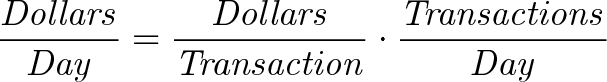$\displaystyle{\mathit{\frac{Dollars}{Day} = \frac{Dollars}{Transaction} \cdot \frac{Transactions}{Day} }}$

Transactions are the workhorse that drive income, but they’re lost in the dollars/day description. When studying an idea, separate the results into Oomph and Often:$\displaystyle{\mathit{ Result = Oomph \cdot Often = \frac{Dollars}{Transaction} \cdot \frac{Transactions}{Day} }}$

With Oomph and Often, I visualize two distinct levers to increase. A ratio like dollars/day makes me stumble through thoughts like: “For better results, I need 1/day to improve… which means the day gets shorter… How’s that possible? Oh, that must be the portion of the day used for each transaction…”.

Why make it difficult? Rewrite the ratio to include the root case: What’s the Oomph, and how Often does it happen?

## Horsepower, Torque, RPM

In physics, we define everyday concepts like “power” with a formal ratio: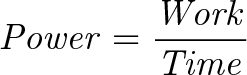$\displaystyle{\mathit{ Power = \frac{Work}{Time} }}$

Ok. Power can be explained by a ratio, but we’re already in inverted-thinking mode. Just another hassle when exploring an already-tricky concept.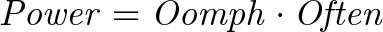$\displaystyle{\mathit{ Power = Oomph \cdot Often }}$

Easier, I think. What could Oomph and Often mean?

Well, Oomph is probably the work we do (such as moving a weight) and Often is how frequently we do it (how many reps did you put in?).

In the same minute, suppose Frank lifted 100lbs ten times, while Annie lifted 1000lbs once. From the equation, they have the same power (though to be honest, I’m more frightened by Annie.)

An engine mechanic might internalize power like this: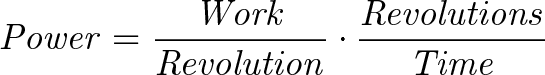$\displaystyle{\mathit{ Power = \frac{Work}{Revolution} \cdot \frac{Revolutions}{Time} }}$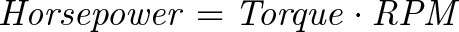$\displaystyle{\mathit{ Horsepower = Torque \cdot RPM }}$

What does that mean?

• Torque is the Oomph, or how much weight (and how far) can be moved by a turn of the engine (i.e., moving 500lbs by 1 foot)

• RPM (revolutions per minute) is how frequently the engine turns

A motorcycle engine is designed for reps, i.e. spinning the wheels quickly. It doesn’t need much torque — just enough to pull itself and a few passengers — but it needs to send that to the wheels again and again.

A bulldozer is designed for “Oomph”, such as knocking over a wall. We don’t need to tap into that work very frequently, as one destroyed wall per minute is great, thanks.

I’m not a physicist or car guy, but I can at least conceptualize the tradeoffs with the Oomph/Often metaphor.

Gears can change the tradeoff between Oomph and Often in a given engine. If you’re going uphill, fighting gravity, what do you want more of? If you’re cruising on a highway? Trying to start from a standstill? Driving over slippery snow? Lost the brakes and need to slow down the car?

Oomph/Often gets me thinking intuitively, Work/Time does not.

## Variation: Electric Power

Electric power has the same ratio as mechanical power: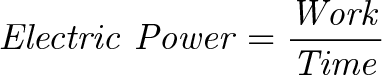$\displaystyle{\mathit{ Electric \ Power = \frac{Work}{Time} }}$

Yikes. It’s not clear what this means. How about: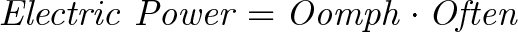$\displaystyle{\mathit{ Electric \ Power = Oomph \cdot Often }}$

It’s hard to have ideas out of the blue, but we might imagine something (a mini-engine?) is moving the Oomph around inside the wire. If we call it a “charge” then we have: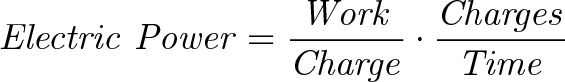$\displaystyle{\mathit{ Electric \ Power = \frac{Work}{Charge} \cdot \frac{Charges}{Time} }}$

And we can give those subparts formal names:

• Voltage (Oomph): How much work each charge contributes

• Current (Often): How quickly charges are moving through the wire

Now we get the familiar: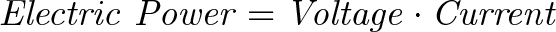$\displaystyle{\mathit{ Electric \ Power = Voltage \cdot Current }}$

Boomshakala! I don’t have a good intuition for electricity, at least my goal is clear: find analogies where voltage means Oomph, and current means Often.

And still, we can take a crack at intuitive thinking: when you get zapped by a doorknob in winter, was that Oomph or Often? What attribute should batteries maximize? What’s better for moving energy through stubborn power lines? (Vive la résistance!)

The ratios think every type of power reduces to a generic Work/Time calculation. The Oomph/Often metaphor gets us thinking about Torque/RPM in one scenario and Voltage/Current in another.

## What’s Really Going On? Parameters, Baby.

The Oomph/Often viewpoint lets us think about the true cause of the ratio. Instead of dollars and days, we wonder how the actual transactions affect the outcome:

• Can we increase the size of each transaction?

• Can we increase the number each day?

In formal terms, we’ve introduced a new parameter to explain the interaction. To change a ratio from a/b to one parameterized by x, we can do: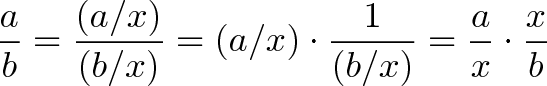$\displaystyle{\frac{a}{b} = \frac{(a/x)}{(b/x)} = (a/x) \cdot \frac{1}{(b/x)} = \frac{a}{x} \cdot \frac{x}{b} }$

We change our viewpoint to see x as the key component. In math, we often switch viewpoints to simplify problems:

• Can we see a giant function as being parameterized by smaller ones? (See the chain rule.)

• Can we express probabilities as odds, instead of percentages? (It makes Bayes Theorem easier.)

Adjusting parameters is a way to morph an idea that doesn’t click into one that does. Since I don’t naturally think with inverted units, I’ve made it easier on myself: deal with two multiplications, instead of a division.

Happy math.